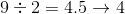# ISEE Lower Level Math : How to find the whole from the part

## Example Questions

← Previous 1 3

### Example Question #86 : Numbers And Operations

Find the whole from the part. Round to the nearest tenths place.

16 is 55% of what number?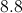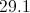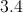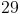Explanation:

To find the whole from a part, divide the "part" by the percent. But first, change the percent into a decimal (55% = 0.55)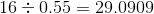Round to the nearest tenths place = 29.1.

### Example Question #7 : Creating Equations With Whole Numbers

Roman is ordering uniforms for the tennis team. He knows how many people are on the team and how many uniforms come in each box. Which equation can be used to solve for how many boxes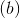Roman should order?

b = number of students ÷ number of uniforms per box

b = number of students x number of uniforms per box

b = number of boxes x number of uniforms per box

b = number of boxes ÷ number of uniforms per box

b = number of students - number of uniforms per box

b = number of students ÷ number of uniforms per box

Explanation:

The total number of uniforms needed equals the number of students divided by the number of uniforms per box.

### Example Question #87 : Numbers And Operations

52 is 25% of what number?

208

1,300

240

13

208

Explanation:

To find the whole from the part, divide the “part” by the percent.  In order to do this, first change the percent into a decimal (25% becomes 0.25).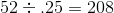Therefore, 52 is 25% of 208.

### Example Question #88 : Numbers And Operations

At a party,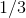of the cake was eaten. If each of the 12 attendees had one piece of cake, how many pieces were in the whole cake?

15

36

4

48

36

Explanation:

12 pieces of cake is equivalent toof the whole. Proportions can be used to determine how many pieces were in the entire cake. Letequal the number of pieces in the entire cake.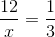Using this set up, both fractions are equal to. Cross multiply.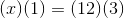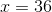### Example Question #89 : Numbers And Operationsisof. Solve for.Explanation: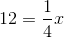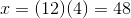### Example Question #1 : How To Find The Whole From The Partis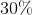of what number?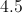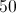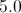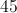Explanation:

Solve by setting up a proportion.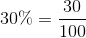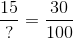Cross multiply.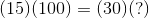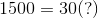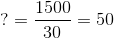isof.

### Example Question #2 : How To Find The Whole From The Part

Which of the following numbers is a multiple of ten?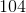Explanation:

A multiple is a number that can be divided by another number without a remainder. 30 can be divided by 10 without a remainder.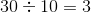It is the only number from the list of answer choices for which this is true. Therefore, it is the correct answer.

### Example Question #3 : How To Find The Whole From The Part

Andrea and her three friends all pitched in to buy a puppy. Each person paid equal parts. If Andrea paid 20 dollars, what was the total cost of the puppy?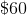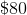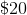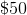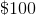Explanation:

We can solve this question with multiplication.

First, we know that Andrea paid 20 dollars and that each person paid equal parts. That means that each person paid 20 dollars.

Second, we know that there are four people total: Andrea and three additional friends.

Multiply the cost per person by the number of people to get the total cost.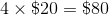### Example Question #4 : How To Find The Whole From The Part

Together, Patricia, Alex and Steve compose 25 percent of their math club. How big is the math club?

9 people

4 people

12 people

10 people

12 people

Explanation:

If 3 people compose 25% of the math club, then 100% of the math club would consist of 4 times the number 3. This gives us a product of 12. Therefore, the correct answer is 12 people.

### Example Question #5 : How To Find The Whole From The Part

Bessy is making shirts for her daughters. If 2 square feet of fabric is enough for her to make 1 shirt, how many shirts can she make with 9 square feet of fabric?

7 shirts

4 shirts

6 shirts

9 shirts

5 shirts